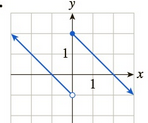Chapter 10.2, Problem 5EFinite Mathematics and Applied Cal...

7th Edition
Stefan Waner + 1 other
ISBN: 9781337274203

Solutions

Chapter
SectionFinite Mathematics and Applied Cal...

7th Edition
Stefan Waner + 1 other
ISBN: 9781337274203
Textbook Problem

In Exercises 1–14 the graph of a function f is given. Determine whether f is continuous on its domain. If it is not continuous on its domain, say why. [HINT: See Quick Examples 1–4.]To determine

Whether the function f is continuous on its domain and give reason if it is not continuous. The graph of function f is as follows:Explanation

Given Information:

The provided graph is:

Consider the provided graph:

From the graph, it is observed that the graph of function f breaks at x=0.

As x is approaching 0 from the left, it is noticed from the graph that y-coordinate approaches 1 means limx0f(x)=1

Still sussing out bartleby?

Check out a sample textbook solution.

See a sample solution

The Solution to Your Study Problems

Bartleby provides explanations to thousands of textbook problems written by our experts, many with advanced degrees!

Get Started

Find more solutions based on key concepts